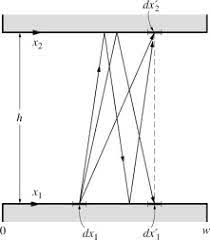## How to Calculate and Solve for Radiosity | Radiation Heat TransferTo compute for radiosity, three essential parameters are needed and these parameters are Total Emissive Power (e), Reflectivity (ρ) and Total Irradiation (G).

J = e + ρG

Where:

e = Total Emissive Power
ρ = Reflectivity

Let’s solve an example;
Find the radiation heat transfer when the total emissive power is 12, the reflectivity is 10 and the total irradiation is 20.

This implies that;

e = Total Emissive Power = 12
ρ = Reflectivity = 10
G = Total Irradiation = 20

J = e + ρG
J = 12 + (10)(20)
J = 12 + 200
J = 212

Therefore, the radiosity is 212 W/m².

Calculating the Total Emissive Power when the Radiosity, the Reflectivity and the Total Irradiation is Given.

e = J – ρG

Where:

e = Total Emissive Power
ρ = Reflectivity

Let’s solve an example;
Find the total emissive power when the radiation heat transfer is 32, the reflectivity is 10 and the total irradiation is 2.

This implies that;

J = Radiation Heat Transfer = 32
ρ = Reflectivity = 10
G = Total Irradiation = 2

e = J – ρG
e = 32 – (10)(2)
e = 32 – 20
e = 12

Therefore, the total emissive power is 12.